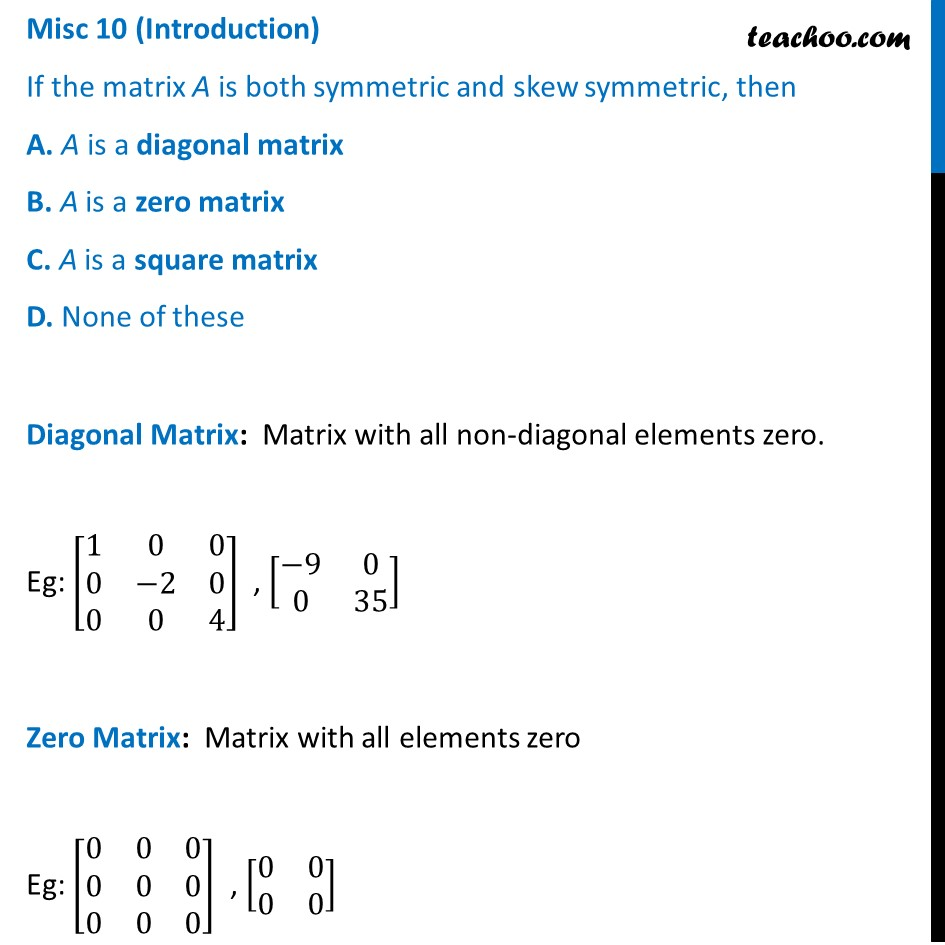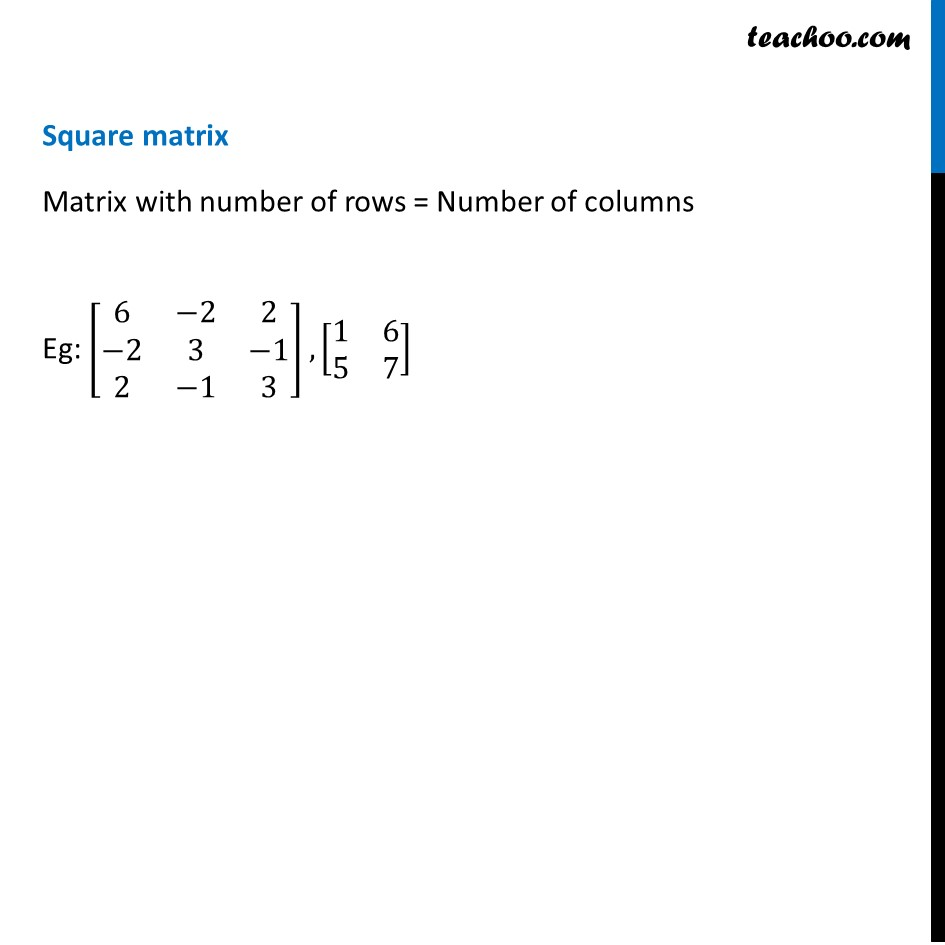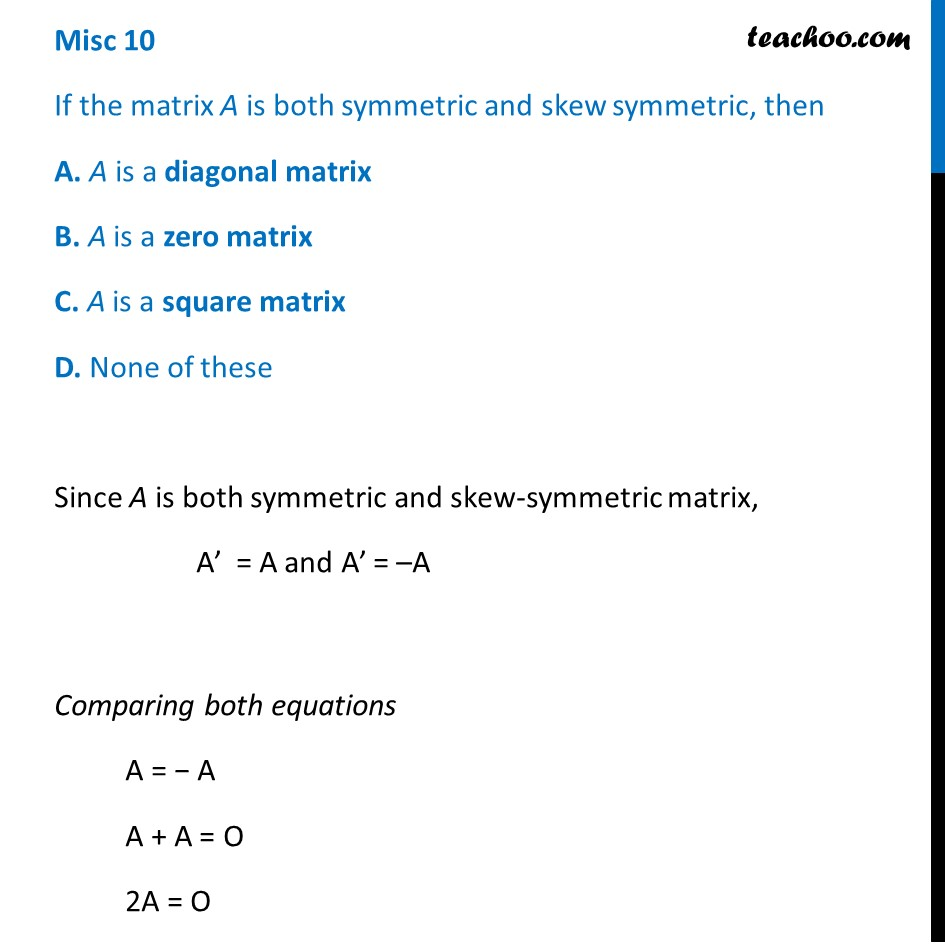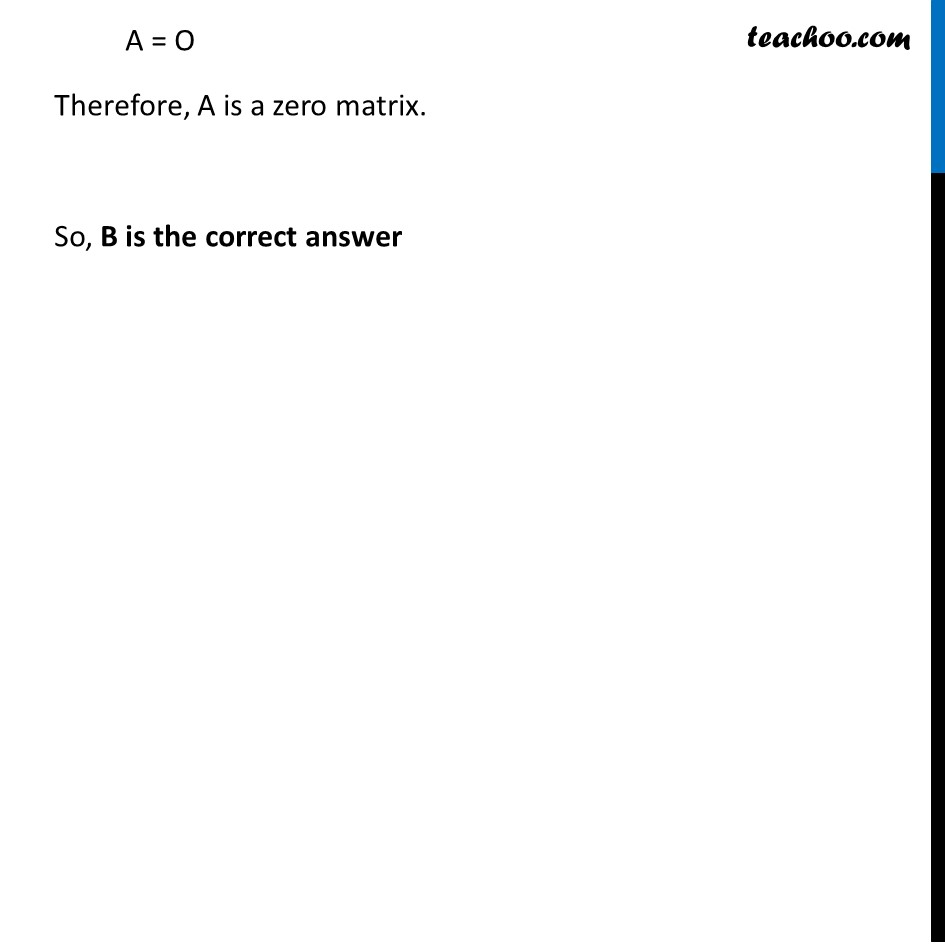Miscellaneous

Chapter 3 Class 12 Matrices
Serial order wiseLearn in your speed, with individual attention - Teachoo Maths 1-on-1 Class

### Transcript

Misc 10 (Introduction) If the matrix A is both symmetric and skew symmetric, then A. A is a diagonal matrix B. A is a zero matrix C. A is a square matrix D. None of these Diagonal Matrix: Matrix with all non-diagonal elements zero. Eg: [■8(1&0&0@0&−2&0@0&0&4)] , [■8(−9&0@0&35)] Zero Matrix: Matrix with all elements zero Eg: [■8(0&0&0@0&0&0@0&0&0)] , [■8(0&0@0&0)] Square matrix Matrix with number of rows = Number of columns Eg: [■8(6&−2&2@−2&3&−1@2&−1&3)] , [■8(1&6@5&7)] Misc 10 If the matrix A is both symmetric and skew symmetric, then A. A is a diagonal matrix B. A is a zero matrix C. A is a square matrix D. None of these Since A is both symmetric and skew-symmetric matrix, A’ = A and A’ = –A Comparing both equations A = − A A + A = O 2A = O A = O Therefore, A is a zero matrix. So, B is the correct answer